# Cramer’s Rule

Cramer's Rule is a technique used to systematically solve systems of linear equations, based on the calculations of determinants.

Typically, solving systems of linear equations can be messy for systems that are larger than 2x2, because there are many ways to go around reducing it when there are three or more variables.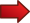Cramer's Rule provide and unequivocal, systematic way of finding solutions to systems of linear equations, no matter the size of the system.The number of calculations required does increase for large systems, but the procedure is exactly the same, regardless of the size of the system.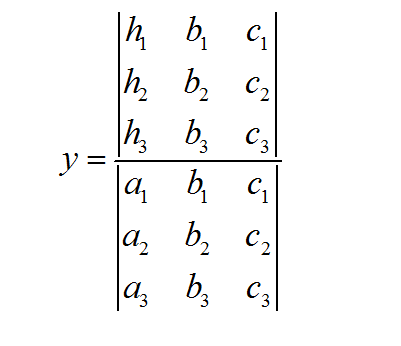## How to Use Cramer's Rule

In order to make things easier, we will work out the case for $$n = 2$$ and then we will establish a more general version which will, hopefully, make better sense after having tackled the $$n=2$$ case.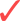Step 1 : All 2x2 linear systems can be written in the following form:

$\large a_1 x + b_1 y = c_1$ $\large a_2 x + b_2 y = c_2$

So then your first step is finding these values $$a_1, b_1, c_1$$ and $$a_2, b_2, c_2$$ for the system you want to solve.Step 2 : Once you have the coefficients $$a_1, b_1, c_1$$ and $$a_2, b_2, c_2$$ you use the following formulas to solve for $$x$$ and $$y$$:

$\large \displaystyle x = \frac{\det \left[\begin{matrix} c_1 & b_1 \\ c_2 & b_2 \end{matrix}\right] }{ \det \left[\begin{matrix} a_1 & b_1 \\ a_2 & b_2 \end{matrix}\right]}$ $\large \displaystyle y = \frac{\det \left[\begin{matrix} a_1 & c_1 \\ a_2 & c_2 \end{matrix}\right] }{ \det \left[\begin{matrix} a_1 & b_1 \\ a_2 & b_2 \end{matrix}\right]}$

In the above formula, where it says "det", it means the determinant of the corresponding matrix. Sometimes, a more compact notation is used for determinants, as it is shown below:

$\large \displaystyle \det \left[\begin{matrix} a_1 & b_1 \\ a_2 & b_2 \end{matrix}\right] = \left|\begin{matrix} a_1 & b_1 \\ a_2 & b_2 \end{matrix}\right|$

So, using the notation above, we would get these more compact formulas for the Cramer's rule:

$\large \displaystyle x = \frac{ \left|\begin{matrix} c_1 & b_1 \\ c_2 & b_2 \end{matrix}\right| }{\left|\begin{matrix} a_1 & b_1 \\ a_2 & b_2 \end{matrix}\right|}$ $\large \displaystyle y = \frac{ \left|\begin{matrix} a_1 & c_1 \\ a_2 & c_2 \end{matrix}\right| }{\left|\begin{matrix} a_1 & b_1 \\ a_2 & b_2 \end{matrix}\right|}$

Let us have a visual way of understanding what is happening. Observe that both $$x$$ and $$y$$ have the same determinant in the denominator.

The coefficients of that common matrix used in the denominator are directly derived from the coefficients that multiply $$x$$ and $$y$$ in the system. See the image below: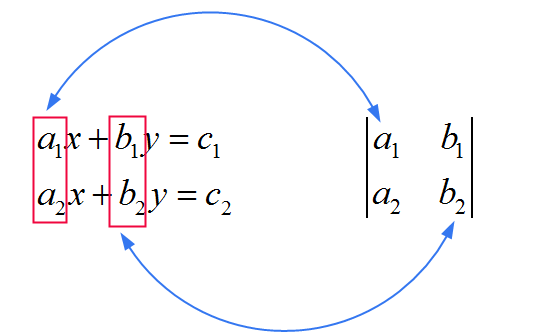Now we see that $$x$$ and $$y$$ differ in what they have in the numerator. As a way of remembering the rule, think of this:For $$x$$, you use the SAME matrix as the one in the denominator, only that you replace the FIRST column with the coefficients $$c_1$$ and $$c_2$$. See the image belowFor $$y$$, you use the SAME matrix as the one in the denominator, only that you replace the SECOND column with the coefficients $$c_1$$ and $$c_2$$. See the image below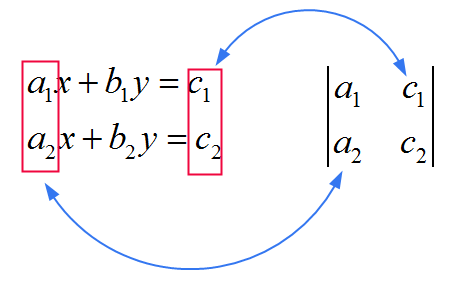### EXAMPLE 1

Solve the following 2x2 linear system:

$\large 2x + 8y = 10$ $\large 2x - 4y = 4$

Let us follow the two steps that we have delineated above to use Cramer's rule to solve the system above:Step 1 : We need to identify the coefficients for the corresponding determinants.

For the matrix that goes in the denominator we use

$\left|\begin{matrix} a_1 & b_1 \\ a_2 & b_2 \end{matrix}\right| = \left|\begin{matrix} 2 & 8 \\ 2 & -4 \end{matrix}\right|$

Now, in this case $$c_1 = 10, c_2 = 4$$, for the determinant used to compute $$x$$, we replace the previous matrix by changing the FIRST column:

$\left|\begin{matrix} c_1 & b_1 \\ c_2 & b_2 \end{matrix}\right| = \left|\begin{matrix} 10 & 8 \\ 4 & -4 \end{matrix}\right|$

For the determinant used to compute $$y$$ we replace the previous matrix by changing the SECOND column:

$\left|\begin{matrix} a_1 & c_1 \\ a_2 & c_2 \end{matrix}\right| = \left|\begin{matrix} 2 & 10 \\ 2 & 4 \end{matrix}\right|$

So now we get the solution:

$\large \displaystyle x = \frac{ \left|\begin{matrix} c_1 & b_1 \\ c_2 & b_2 \end{matrix}\right| }{\left|\begin{matrix} a_1 & b_1 \\ a_2 & b_2 \end{matrix}\right|}$ $= \frac{\left|\begin{matrix} 10 & 8 \\ 4 & -4 \end{matrix}\right|}{\left|\begin{matrix} 2 & 8 \\ 2 & -4 \end{matrix}\right|} = \frac{10 \times (-4) - 4\times 8}{2 \times (-4) - 2 \times 8} = \frac{-72}{-24} = 3$

and for $$y$$:

$\large \displaystyle y = \frac{ \left|\begin{matrix} a_1 & c_1 \\ a_2 & c_2 \end{matrix}\right| }{\left|\begin{matrix} a_1 & b_1 \\ a_2 & b_2 \end{matrix}\right|}$ $= \frac{\left|\begin{matrix} 2 & 10 \\ 2 & 4 \end{matrix}\right|}{\left|\begin{matrix} 2 & 8 \\ 2 & -4 \end{matrix}\right|} = \frac{2 \times 4 - 2\times 10}{2 \times (-4) - 2 \times 8} = \frac{-12}{-24} = \frac{1}{2}$

Therefore, the solution is $$x = 3$$, $$y = 1/2$$.

## Cramer's Rule for the General Case

The beauty of Cramer's rule is that it applies exactly the same procedure, whether it is a 2x2 system or if it is a 10x10 system. The concept is the same.

So, assume that $$x_1, x_2, ..., x_n$$ are the variables (the unknowns), and we want to solve the following n x n system of linear equations:

$\large a_{11} x_1 + a_{12} x_2 + .... + a_{1n} x_n = c_1$ $\large a_{21} x_1 + a_{22} x_2 + .... + a_{2n} x_n = c_2$ $\large \vdots$ $\large a_{n1} x_1 + a_{n2} x_2 + .... + a_{nn} x_n = c_n$

In order to solve for $$x_1, x_2, ..., x_n$$, we will use the following determinant on the denominator:

$\large\displaystyle \left|\begin{matrix} a_{11} & a_{12} & ... & a_{1n} \\ a_{21} & a_{22} & ... & a_{2n} \\ \vdots & \vdots & ... & \vdots\\ a_{n1} & a_{n2} & ... & a_{nn}\end{matrix}\right|$

• The solution for $$x_1$$ is

$\large\displaystyle x_1 = \frac{ \left|\begin{matrix} c_1 & a_{12} & ... & a_{1n} \\ c_2 & a_{22} & ... & a_{2n} \\ \vdots & \vdots & ... & \vdots\\ c_n & a_{n2} & ... & a_{nn}\end{matrix}\right| }{\left|\begin{matrix} a_{11} & a_{12} & ... & a_{1n} \\ a_{21} & a_{22} & ... & a_{2n} \\ \vdots & \vdots & ... & \vdots\\ a_{n1} & a_{n2} & ... & a_{nn}\end{matrix}\right|}$

• The solution for $$x_2$$ is

$\large\displaystyle x_1 = \frac{ \left|\begin{matrix} a_{11} & c_1 & ... & a_{1n} \\ a_{21} & c_2 & ... & a_{2n} \\ \vdots & \vdots & ... & \vdots\\ a_{n1} & a_{n2} & ... & c_n \end{matrix}\right| }{\left|\begin{matrix} a_{11} & a_{12} & ... & a_{1n} \\ a_{21} & a_{22} & ... & a_{2n} \\ \vdots & \vdots & ... & \vdots\\ a_{n1} & a_{n2} & ... & a_{nn}\end{matrix}\right|}$

And so on. As you can see, the determinant in the denominator is the same, and the one in the numerator is obtained by changing the first column with $$(c_1, ..., c_n)$$ for $$x_1$$. For $$x_2$$ we change the second column by $$(c_1, ..., c_n)$$, for $$x_3$$ we change the third column, and so on. You get the idea.

### EXAMPLE 2

Solve the following system of 3x3 linear equations using Cramer's Rule.

$\large x_1 + x_2 + x3 = 20$ $\large x_1 - x_2 + x3 = 4$ $\large 2x_1 + x_2 - x3 = 16$

First of all, we identify the determinant that goes in the denominator:

$\large\displaystyle \left|\begin{matrix} 1 & 1 & 1 \\ 1 & -1 & 1 \\ 2 & 1 & -1 \end{matrix}\right|$

Also, we need to identify the vector of $$c_i$$ coefficients:

$\large\displaystyle \left[\begin{matrix} 20 \\ 4 \\ 16 \end{matrix}\right]$

This vector will be the one that will be replacing the corresponding columns of the common determinant from the denominator. We get:

$\large\displaystyle x_1 = \frac{ \left|\begin{matrix} 20 & 1 & 1 \\ 4 & -1 & 1 \\ 16 & 1 & -1 \end{matrix}\right| }{ \left|\begin{matrix} 1 & 1 & 1 \\ 1 & -1 & 1 \\ 2 & 1 & -1 \end{matrix}\right| } = \frac{40}{6} = \frac{20}{3}$ $\large\displaystyle x_2 = \frac{ \left|\begin{matrix} 1 & 20 & 1 \\ 1 & 4 & 1 \\ 2 & 16 & -1 \end{matrix}\right| }{ \left|\begin{matrix} 1 & 1 & 1 \\ 1 & -1 & 1 \\ 2 & 1 & -1 \end{matrix}\right| } = \frac{40}{6} = \frac{20}{3}$ $\large\displaystyle x_3 = \frac{ \left|\begin{matrix} 1 & 1 & 20 \\ 1 & -1 & 4 \\ 2 & 1 & 16 \end{matrix}\right| }{ \left|\begin{matrix} 1 & 1 & 1 \\ 1 & -1 & 1 \\ 2 & 1 & -1 \end{matrix}\right| } = \frac{40}{6} = \frac{20}{3}$

Cramer's Rule has a specific role in efficiently solving systems of linear equations. It involves the use of determinants to make very straightforward a task that otherwise would be really complicated, especially for larger systems.

Ultimately, in order to solve linear systems , all you have to do is to identify a number matrix determinants based on the system that needs to be solved, and the conduct a simple algebraic operation to solve the system.

### Applications

Cramer's rule has many applications in both Linear Algebra and Differential Equations.